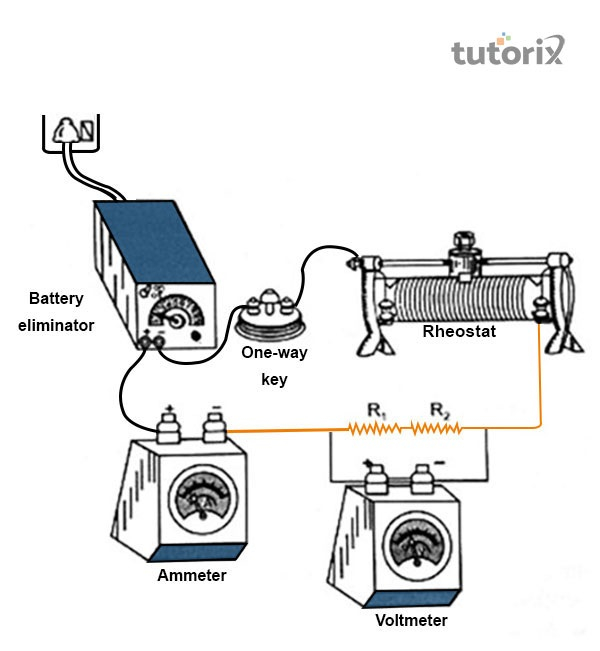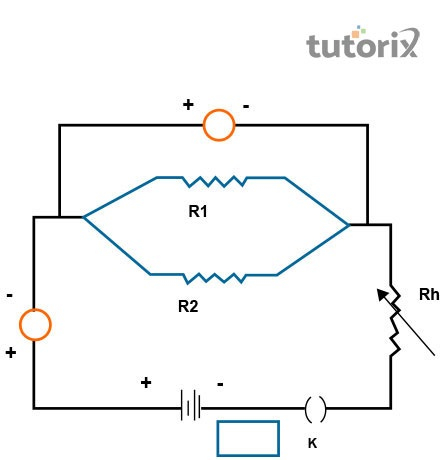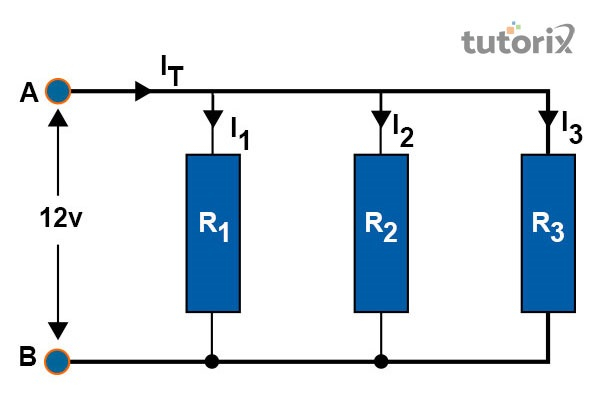# To determine equivalent resistance of resistors when connected in series and in parallel

## Introduction

A circuit is the most important factor here and it works during the time of connecting the resistors in a parallel situation. It is seen that the potential difference in parallel circuit remains the same for every resistor but the flow of current is not the same within the resistor. The calculation of the total current flow is done by swimming up the flow of currents through each resister.

The applications of the parallel circuits have been seen in the household electric distribution. This kind of circuit is preferred for application for the avoidance of short circuits and it helps in monitoring the flow of current for different devices.

## Aim

The main aim of these two factors is to give a determination of the equivalent resistance of these resistors when they are connected in parallel.

## Theory

It is seen that when more than two or two resistors are connected together between the two points, then the situation is called connected in parallel. In the combination process of a parallel situation, the difference of the potentials remains the same across each other (Brainigniter, 2022). Another important aspect of this matter is that the currents which flow through the resistor seem different. The formula shows that:

$$\mathrm{1/R_p = 1/R_1 \:+\: 1/R_2 \:+\: 1/R_3}$$

## Required Materials

There are different required materials for this examination of the factor. Among the required materials there are- two resistors, ammeter, a battery eliminator, required plug key, a piece of sandpaper, wires for connection, and rheostat and a voltmeter (Betrained, 2022). These are considered important materials for the examination process.Figure 1: Aperture arrangement

## Circuit Diagrams and Procedure

At the very beginning, a device will have to be set up as it is shown in diagram (i) of the circuit. After that, one has to plug the key and will have to adjust the rheostat. The adjustment will be continued until a reading is shown by the voltmeter and ammeter and it is done through sliding the variables (Yang et al. 2019).

Then the readings will be taken off the ammeter and voltmeter and the battery is then switched off for a while of the moment. In the next diagrams, the steps are repeated. The resistance is calculated using the formula R = V/1. There are the stages through which the calculation is done of the diagrams.Figure 2: Circuit Diagrams

## Observation Table

### Table for $\mathrm{R_1}$:

1.0.02 V0.01 A2 $\mathrm{\Omega}$
2.0.04 V0.02 ARemains the same
3.0.08 V0.04 ARemains the same

Table 1: $\mathrm{R_1}$ value calculation

Here, the mean value of $\mathrm{R_1 = (2+2+2)/3 = 2\:\Omega}$

### Table for $\mathrm{R_2}$:

1.0.01 V0.01 A1 $\mathrm{\Omega}$
2.0.02 V0.02 A1 $\mathrm{\Omega}$
3.0.04 V0.04 A1 $\mathrm{\Omega}$

Table 2: $\mathrm{R_2}$ value calculation

Here, the mean value of $\mathrm{R_2 = (1+1+1)/3 = 1\:\Omega}$

### Table for $\mathrm{R_p}$:

1.0.02 V0.03 A2/3 $\mathrm{\Omega}$
2.0.04 V0.06 A2/3 $\mathrm{\Omega}$
3.0.06 V0.09 A2/3 $\mathrm{\Omega}$

Table 3: $\mathrm{R_3}$ value calculation

Here, the mean value of $\mathrm{R_p=(2/3+2/3+2/3)/3 = 2/3\:\Omega = 0.67\:\Omega}$

## Result

The result of this matter is that the value that is calculated of the equivalent resistance $\mathrm{(R_p)=2/3\:\Omega\:=\:0.67\:\Omega}$ The value comes from an experiment of the equivalent resistance $\mathrm{(R_p)=2/3\:\Omega\:=\:0.67\:\Omega}$ Ω (Electronics, 2022). The most important factor here is that the $\mathrm{R_p}$ is less than the individual resistances.Figure 3: Resistors in Parallel

## Precautions

This process needs to have some precautions in the matter. It says that thick copper wires should be more effective than the connecting wires. The connections of all the wires should have to be tight in this case (Helseth, 2019). It is important to be precautious about the connection of the voltmeter and the connection should be in parallel with the resistor. The flow of the current should have to be for a short time, for avoiding the unnecessary heating of the circuit.

## Conclusion

The idea here is the concept of the parallel circuit and series of circuits. Here the voltmeter is connected in a matter of parallel and it is done when the resistors are in series. The maximum result in this matter can be get using the resultant resistant. The most important point here is that the resistors should be connected in series. The connectors of the voltmeter say that connections should be as per the set up of the experimental setup.

Q1. What is the concept of equivalent resistance in a series circuit?

Ans: It is seen that the equivalent resistance is the algebraic sum of the resistance. The Law of Ohm helps to find the flow of current within the circuit. It is equal to the voltage and it is divided through the equivalent resistance.

Q2. What is the concept of the equivalent of two resistors in a joined series?

Ans: It is seen that when the two equal resistors are connected in series, then the total resistance of the series will be R + R = 2R. The concept of the joined series is depicted through the formula given in the above point.

Q3. Why does resistance differ in parallel and series?

Ans: The concept differs from each other because parallel circuit has different characteristics. It is also seen that the total resistance also differs from the sum of the resistors.